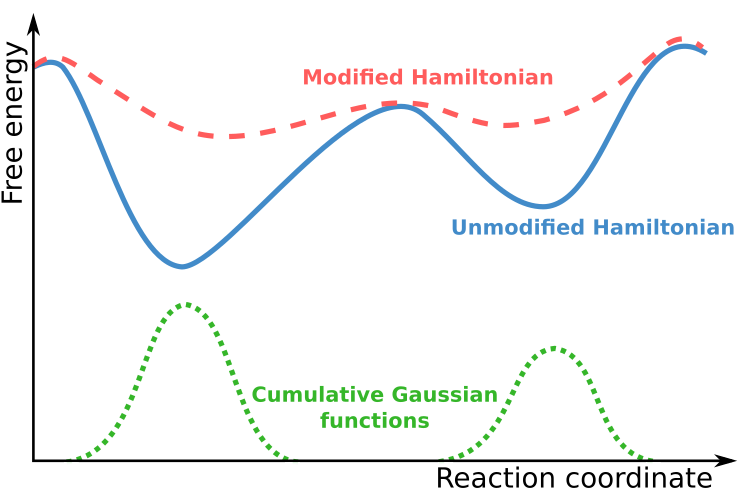### Introduction

Metadynamics is an enhanced sampling method and is informally described as "filling the free energy wells with computational sand".In Metadynamics simulations, the system is described using a set of collective variables that define transitions along a reaction coordinate. During the simulation, the location of the system in this collective variable space is determined, and positive biasing Gaussian functions are added to the overall potential, thus modifying the Hamiltonian of the system.

$$H = T + V + \sum V_\textrm{GAUSS}$$

The accumulation of these Gaussian functions in well sampled regions of the collective variable space results in a flattening of the free energy surface, thereby allowing the system to more easily traverse regions of collective variable space that correspond to free energy maxima when simulating the unmodified Hamiltonian. This allows the simulation to explore the full energy landscape. With knowledge of the sampling of the modified Hamiltonian, and the deposited Gaussian functions, it is possible to recover the free energy surface of the unmodified Hamiltonian.

### Computational Tools

Metadynamics can be performed using the following software tools:

### Example Cases

For examples of previously performed studies in which Metadynamics was the primary method used, see the following example cases:

Metadynamics was also used in the following examples: